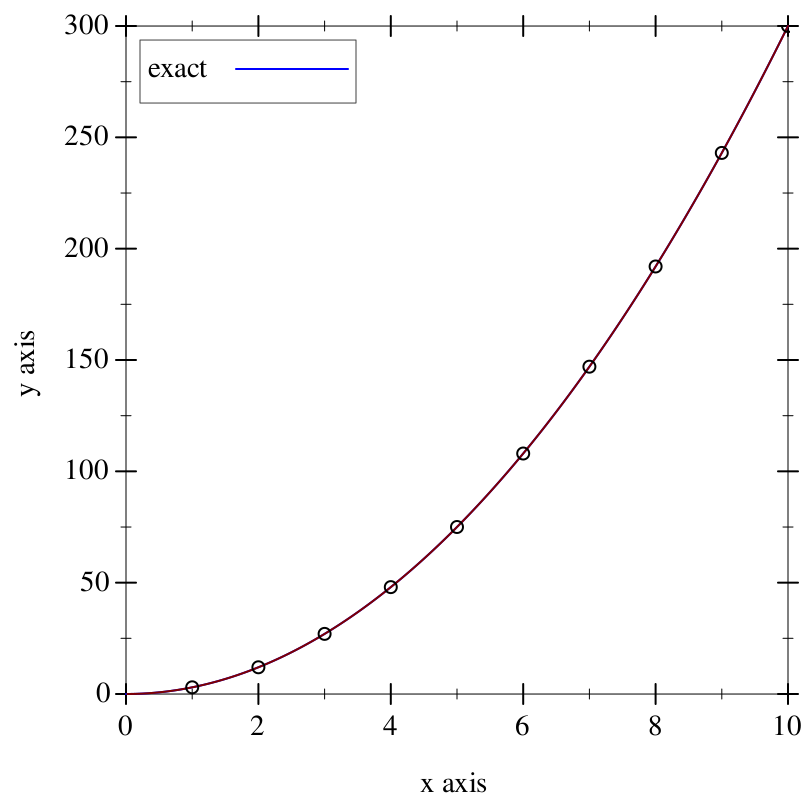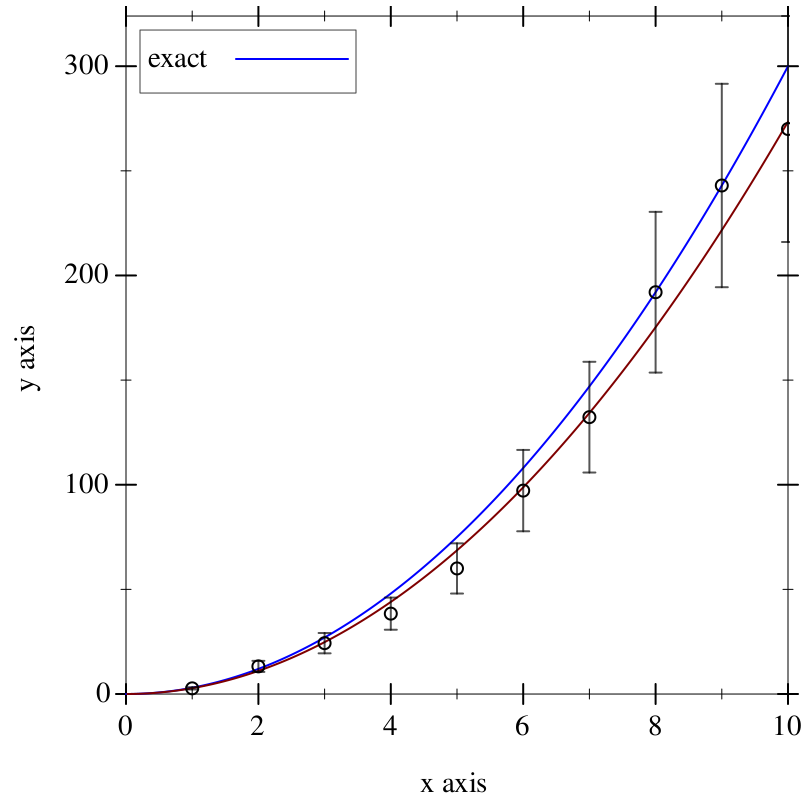8.3

## Bestfit: Lines of Best Fit

 (require bestfit) package: plot-bestfit

Bestfit is a library for calculating lines of best fit using Least Squares Fitting.

 procedure(graph/linear xs ys [errors]) → (Values renderer2d? renderer2d? renderer2d?) xs : (Listof Nonnegative-Flonum) ys : (Listof Nonnegative-Flonum) errors : (U #f (Listof Flonum)) = #f
 procedure(graph/exponential xs ys [errors]) → (Values renderer2d? renderer2d? renderer2d?) xs : (Listof Nonnegative-Flonum) ys : (Listof Nonnegative-Flonum) errors : (U #f (Listof Flonum)) = #f
 procedure(graph/log xs ys [errors]) → (Values renderer2d? renderer2d? renderer2d?) xs : (Listof Nonnegative-Flonum) ys : (Listof Nonnegative-Flonum) errors : (U #f (Listof Flonum)) = #f
 procedure(graph/power xs ys [errors]) → (Values renderer2d? renderer2d? renderer2d?) xs : (Listof Nonnegative-Flonum) ys : (Listof Nonnegative-Flonum) errors : (U #f (Listof Flonum)) = #f
Uses linear-fit, exp-fit, log-fit, power-fit, to generate three renderer2d?s: A plot of the points given by xs and ys, a plot of the function of best fit, and error bars generated. The error bars are generated from error, which is the percentage error on each y coordinate.

> (define (3x^2 x) (* 3.0 (expt x 2.0)))
> (define (add-error y) (+ y (* y (/ (- (random 4) 2) 10.0))))
> (define exact (function 3x^2 #:label "exact" #:color "blue"))
 > (define-values (pts fit _) (graph/power (build-list 10 (compose fl add1)) (build-list 10 (compose 3x^2 fl add1))))
> (plot (list exact fit pts))> (define-values (pts fit err) (graph/power (build-list 10 (compose fl add1)) (build-list 10 (compose add-error 3x^2 fl add1)) (build-list 10 (const 0.2))))
> (plot (list exact fit pts err))procedure(linear-fit xs ys) → (-> Nonnegative-Flonum Real) xs : (Listof Nonnegative-Flonum) ys : (Listof Nonnegative-Flonum)
 procedure(exp-fit xs ys) → (-> Nonnegative-Flonum Real) xs : (Listof Nonnegative-Flonum) ys : (Listof Nonnegative-Flonum)
 procedure(log-fit xs ys) → (-> Nonnegative-Flonum Real) xs : (Listof Nonnegative-Flonum) ys : (Listof Nonnegative-Flonum)
 procedure(power-fit xs ys) → (-> Nonnegative-Flonum Real) xs : (Listof Nonnegative-Flonum) ys : (Listof Nonnegative-Flonum)

Uses Least Squares Fitting to generate a best fit function of the given type.

 > (define line (linear-fit '(1.0 2.0 3.0) '(1.0 2.0 3.0))) > (line 10.0) 10.0 > (line 12.0) 12.0

 procedure(linear-fit-params xs ys) → (Values Real Real) xs : (Listof Nonnegative-Flonum) ys : (Listof Nonnegative-Flonum)
Returns values a and b used to generate a best fit function of the form y = a + b x.

> (linear-fit-params '(1.0 2.0 3.0) '(2.0 4.0 6.0))
 0 2

 procedure(exp-fit-params xs ys) → (Values Real Real) xs : (Listof Nonnegative-Flonum) ys : (Listof Nonnegative-Flonum)
Returns values A and B used to generate a best fit function of the form y = A e^(B x).

> (exp-fit-params '(1.0 2.0 3.0) '(2.0 4.0 8.0))
 1 0.693147

 procedure(log-fit-params xs ys) → (Values Real Real) xs : (Listof Nonnegative-Flonum) ys : (Listof Nonnegative-Flonum)
Returns values a and b used to generate a best fit function of the form y = a + b ln(x).

> (log-fit-params '(2.0 4.0 8.0) '(1.0 2.0 3.0))
 1.83333 0.120225

 procedure(power-fit-params xs ys) → (Values Real Real) xs : (Listof Nonnegative-Flonum) ys : (Listof Nonnegative-Flonum)
Returns values A and B used to generate a best fit function of the form y = A x^B.

> (power-fit-params '(1.0 2.0 3.0) '(2.0 8.0 18.0))
 0.693147 2